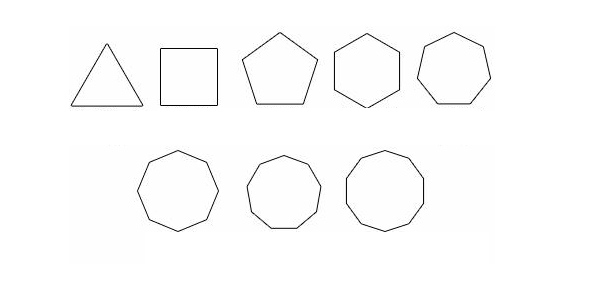# Quiz 4/29

15 Questions | Total Attempts: 79SettingsThis is your weekly quiz for April 29 which is a review or many of the formulas we have used this semester and some new material. You many use your formulas review powerpoint from earlier this week to help you on the quiz.

Related Topics
• 1.
What is the area of the polygon shown below?
• A.

75 cm^2

• B.

21.25 cm^2

• C.

30 cm^2

• D.

12.5 cm^2

• 2.
What is the area of the polygon below?
• A.

36 cm^2

• B.

18 cm^2

• C.

13 cm^2

• D.

26 cm^2

• 3.
What is the perimeter of the regular polygon below?
• A.

3 in

• B.

9 in

• C.

15 in

• D.

18 in

• 4.
What is the area of the regular hexagon below?
• A.

374.04

• B.

72

• C.

124.68

• D.

62.34

• 5.
What is the area of the polygon below?
• A.

50

• B.

80

• C.

40

• D.

25

• 6.
What is the perimeter of the square below?
• A.

X

• B.

4x

• C.

X^2

• D.

None of the above

• 7.
What is the area of the circle below?
• A.

194.68 ft^2

• B.

97.34 ft^2

• C.

3017.54 ft^2

• D.

12070.16 ft^2

• 8.
What is the circumference of the circle below?
• A.

40.82 in

• B.

81.64 in

• C.

530.66 in

• D.

2122.64 in

• 9.
What is the midpoint of these two points: (4, 8) and (7, 12)?
• A.

(-3, -4)

• B.

(11, 20)

• C.

(-1.5, -2)

• D.

(5.5, 10)

• 10.
What is the distance between the two points: (8, 14) and (5, -7)?
• A.

14.76

• B.

21.21

• C.

13.42

• D.

None of the above

• 11.
What is the product of the two fractions (3/4) and (1/2)?
• A.

4/6

• B.

3/8

• C.

5/4

• D.

6/4

• 12.
What is the least common denominator for the following fractions: (5/36), (5/6), and (5/9)?
• A.

5

• B.

3

• C.

12

• D.

36

• 13.
As a police officer you spent 189 minutes monitoring a public even.  What is this time expressed in hours and minutes?
• A.

2 hours and 9 minutes

• B.

2 hours and 89 minutes

• C.

3 hours and 9 minutes

• D.

1 hour and 89 minutes

• 14.
In your job as a carpenter, you are building a cabinet.  The door of the cabinet is 3/4 inch thick.  The trim on the door is 1/2 inch thick.  How many total inche(es) thick are the door and the trim together?
• A.

1/2

• B.

1 1/4

• C.

4/6

• D.

1 1/2

• 15.
At the drugstore that you work at, an 8-ounce bottle of Champion Shampoo sells for \$2.39.  A 6-ounce bottle of Foamy Bubbles Shampoo sells for \$3.59.  If you want to make a sign advertising the price per ounce of the better deal, what price per ounce would the sign report?
• A.

0.4 ¢

• B.

29.9 ¢

• C.

39.8 ¢

• D.

59.8 ¢